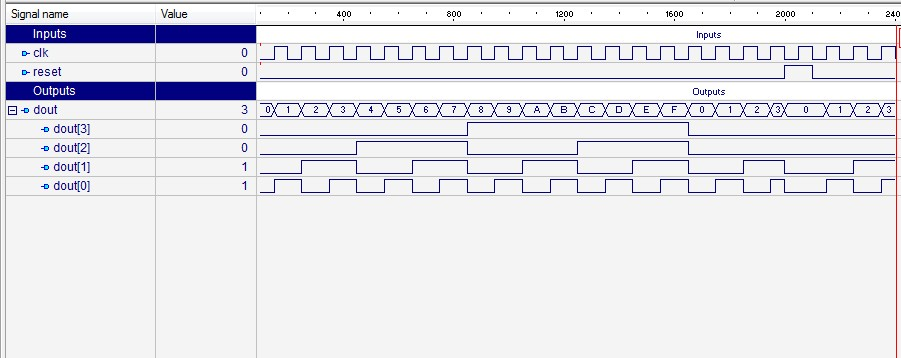Monday, 22 July 2013

Design of 4 Bit Binary Counter using Behavior Modeling Style (VHDL Code).

Design of 4 Bit Binary Counter using Behavior Modeling Style -Output Waveform :   4 Bit Binary Counter

VHDL Code -

-------------------------------------------------------------------------------
--
-- Title       : counter_4bit
-- Design      : vhdl_upload2
-- Author      : Naresh Singh Dobal
-- Company     : nsdobal@gmail.com
-- VHDL Programs &  Exercise with Naresh Singh Dobal.
--
-------------------------------------------------------------------------------
--
-- File        : Design of 4 bit counter using behavior modeling style.vhd

library IEEE;
use IEEE.STD_LOGIC_1164.all;
use IEEE.STD_LOGIC_ARITH.all;
use IEEE.STD_LOGIC_UNSIGNED.all;

entity counter_4bit is
port(
clk : in STD_LOGIC;
reset : in STD_LOGIC;
dout : out STD_LOGIC_VECTOR(3 downto 0)
);
end counter_4bit;

architecture counter_4bit_arc of counter_4bit is
begin

counting : process (clk,reset) is
variable m : std_logic_vector (3 downto 0) := "0000";
begin
if (reset='1') then
m := "0000";
elsif (rising_edge (clk)) then
m := m + 1;
end if;
dout <= m;
end process counting;

end counter_4bit_arc;

Design by Wordpress Theme | Bloggerized by Free Blogger Templates | coupon codes